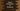# Python Dictionary introduction : Python Tutorial : Part 11## Python Dictionary: Introduction:

In this tutorial, we will discuss Python dictionary and some built-in methods. A dictionary is a collection of_ (key: value)_ paired items. All elements of a dictionary are placed inside curly braces { }, each element is separated by a comma. The elements of a dictionary are unordered and using a key, we can get a value.

All keys should be unique in a dictionary and should be of immutable data types.

## Create a Python dictionary:

We can create a dictionary in python using curly braces directly or by using dict() method.

``````dict1 = { "one":1 , "two":2 ,"three":3 }
print dict1 #{'three': 3, 'two': 2, 'one': 1}

dict2 = {}
print dict2 # {}

dict3 = dict({"one":1 ,"two":2 ,"three":3 })
print dict3 # {'one': 1, 'three': 3, 'two': 2}

dict4 = dict([("one",1),("two",2),("three",3)])
print dict4 # {'three': 3, 'two': 2, 'one': 1}``````

In the above example, dict2 is an empty dictionary.

## Access an element of a python dictionary:

Since keys are unique, using a key we can get the value of a dictionary element.Using a square bracket, and passing the key, we can get the value for that key. If the key is not in the dictionary, it will throw a key error. We can also use get() instead of using a square bracket. get() doesn’t throw any key error.

``````dict1 = { "one" : 1, "two" : 2, "three" : 3, "four" : 4}
print dict1["one"] # 1

# print dict1["five"] #key error

print dict1.get("four") # 4

print dict1.get("five") # Prints None``````

## Updating and Deleting dictionary elements:

Python dictionary is mutable. We can add or update a value inside a dictionary. Using the key, we can update its value or we can add a whole key-value pair to a dictionary as shown below:

``````dict1 = { "one" : 1, "two" : 2, "three" : 3, "four" : 4}
print dict1 # {'four': 4, 'three': 3, 'two': 2, 'one': 1}

dict1["four"] = 4.0
print dict1 # {'four': 4.0, 'three': 3, 'two': 2, 'one': 1}

dict1["five"] = 5
print dict1 # {'four': 4.0, 'three': 3, 'five': 5, 'two': 2, 'one': 1}``````

## To delete an element of a dictionary:

We can delete a specific item of a dictionary or we can delete the complete dictionary. ‘del()’ statement is used mainly for this. Also, ‘clear()’ and ‘pop()’ can be used. ‘pop()’ returns the element that is removed and clear() removes all elements of a dictionary.

``````dict1 = { "one" : 1, "two" : 2, "three" : 3, "four" : 4}
print dict1 # {'four': 4, 'three': 3, 'two': 2, 'one': 1}

del dict1["four"]
print dict1 # {'three': 3, 'two': 2, 'one': 1}

print dict1.pop("three") # 3

print dict1 # {'two': 2, 'one': 1}

dict1.clear()
print dict1 # {}

del dict1
print dict1 # Name error will be thrown``````

## Iterating through a python dictionary:

Using a for loop, we can iterate through a python dictionary.

``````dict1 = { "one" : 1, "two" : 2, "three" : 3, "four" : 4}

for i in dict1 :
print dict1[i]``````

The above program will print all values of the dictionary dict1.

That’s all for python dictionary. In our next tutorial, we will check different commonly used dictionary methods.